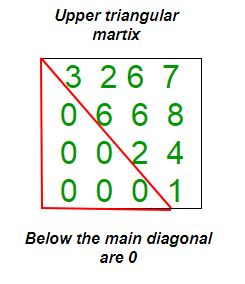Open In App

# C++ Program to check if matrix is upper triangular

Given a square matrix and the task is to check the matrix is in upper triangular form or not. A square matrix is called upper triangular if all the entries below the main diagonal are zero.Examples:

```Input : mat = {{1, 3, 5, 3},
{0, 4, 6, 2},
{0, 0, 2, 5},
{0, 0, 0, 6}};
Output : Matrix is in Upper Triangular form.

Input : mat = {{5, 6, 3, 6},
{0, 4, 6, 6},
{1, 0, 8, 5},
{0, 1, 0, 6}};
Output : Matrix is not in Upper Triangular form.```

## C++

 `// Program to check upper triangular matrix.``#include ``#define N 4``using` `namespace` `std;` `// Function to check matrix is in upper triangular``// form or not.``bool` `isUpperTriangularMatrix(``int` `mat[N][N])``{``    ``for` `(``int` `i = 1; i < N; i++)``        ``for` `(``int` `j = 0; j < i; j++)``            ``if` `(mat[i][j] != 0)``                ``return` `false``;``    ``return` `true``;``}` `// Driver function.``int` `main()``{``    ``int` `mat[N][N] = { { 1, 3, 5, 3 },``                      ``{ 0, 4, 6, 2 },``                      ``{ 0, 0, 2, 5 },``                      ``{ 0, 0, 0, 6 } };``    ``if` `(isUpperTriangularMatrix(mat))``        ``cout << ``"Yes"``;``    ``else``        ``cout << ``"No"``;``    ``return` `0;``}`

Output:

`Yes`

Time Complexity: O(n2), where n represents the number of rows and columns of the matrix.
Auxiliary Space: O(1), no extra space is required, so it is a constant.

Please refer complete article on Program to check if matrix is upper triangular for more details!